Updating search results...

# 28 Results

View
Selected filters:
• Wisc-Online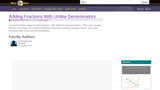Restricted Use
Rating
0.0 stars

Learners follow steps to add fractions with different denominators. They also review fraction concepts, do a drag-and-drop exercise involving fraction terms, and add fractions with common denominators.

Subject:
Mathematics
Material Type:
Interactive
Provider:
Wisc-Online
12/23/2015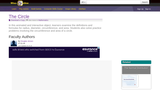Restricted Use
Rating
0.0 stars

In this animated and interactive object, learners examine the definitions and formulas for radius, diameter, circumference, and area. Students also solve practice problems involving the circumference and area of a circle.

Subject:
Mathematics
Material Type:
Interactive
Provider:
Wisc-Online
12/23/2015Restricted Use
Rating
0.0 stars

Students recognize the conversions used among fractions, decimals, and percents

Subject:
Mathematics
Material Type:
Interactive
Provider:
Wisc-Online
12/23/2015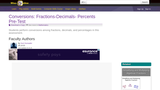Restricted Use
Rating
0.0 stars

Students perform conversions among fractions, decimals, and percentages in this assessment.

Subject:
Mathematics
Material Type:
Interactive
Provider:
Wisc-Online
12/23/2015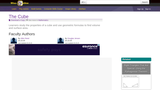Restricted Use
Rating
0.0 stars

Learners study the properties of a cube and use geometric formulas to find volume and surface area.

Subject:
Mathematics
Material Type:
Interactive
Provider:
Wisc-Online
12/23/2015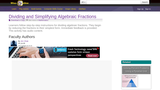Restricted Use
Rating
0.0 stars

Learners follow step-by-step instructions for dividing algebraic fractions. They begin by reducing the fractions to their simplest form. Immediate feedback is provided. This activity has audio content.

Subject:
Mathematics
Material Type:
Interactive
Provider:
Wisc-Online
12/23/2015Restricted Use
Rating
0.0 stars

The learner factors an algebraic expression of the form AX(Squared) + BX + C, where the coefficient of the X squared term is not equal to 1.

Subject:
Mathematics
Material Type:
Interactive
Provider:
Wisc-Online
12/23/2015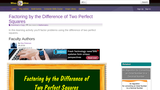Restricted Use
Rating
0.0 stars

In this interactive object, students work problems using the difference of two perfect squares.

Subject:
Mathematics
Material Type:
Interactive
Provider:
Wisc-Online
12/23/2015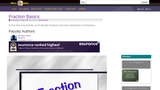Restricted Use
Rating
0.0 stars

Students view illustrations of fractions and identify fractions.

Subject:
Mathematics
Material Type:
Interactive
Provider:
Wisc-Online
12/23/2015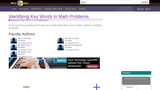Restricted Use
Rating
0.0 stars

Students read lists of the key words that direct them to add, subtract, multiply, or divide. They then identify them in an interactive exercise.

Subject:
Mathematics
Material Type:
Interactive
Provider:
Wisc-Online
12/23/2015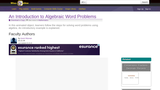Restricted Use
Rating
0.0 stars

In this animated object, learners follow the steps for solving word problems using algebra. An introductory example is explained.

Subject:
Mathematics
Material Type:
Interactive
Provider:
Wisc-Online
12/23/2015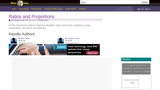Restricted Use
Rating
0.0 stars

In this interactive object, learners simplify ratios and solve problems using proportions. All terms are defined.

Subject:
Mathematics
Material Type:
Interactive
Provider:
Wisc-Online
12/23/2015Restricted Use
Rating
0.0 stars

Learners study the properties of a rectangular solid and use geometric formulas to find volume and surface area.

Subject:
Mathematics
Material Type:
Interactive
Provider:
Wisc-Online
12/23/2015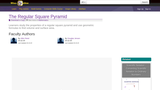Restricted Use
Rating
0.0 stars

Students will use the geometric formula for finding the surface area of a regular square pyramid.

Subject:
Mathematics
Material Type:
Interactive
Provider:
Wisc-Online
12/23/2015Restricted Use
Rating
0.0 stars

Learners study the properties of a right circular cone and use geometric formulas to find volume and surface area.

Subject:
Mathematics
Material Type:
Interactive
Provider:
Wisc-Online
12/23/2015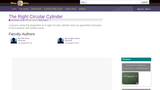Restricted Use
Rating
0.0 stars

Learners study the properties of a right circular cylinder and use geometric formulas to find volume and surface area.

Subject:
Mathematics
Material Type:
Interactive
Provider:
Wisc-Online
12/23/2015Restricted Use
Rating
0.0 stars

The learner reads an explanation of scientific notation and practices expressing numbers larger than 1 in scientific notation.

Subject:
Mathematics
Material Type:
Interactive
Provider:
Wisc-Online
12/23/2015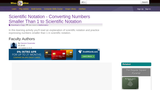Restricted Use
Rating
0.0 stars

The student reads an explanation of scientific notation and practices expressing numbers smaller than 1 in scientific notation.

Subject:
Mathematics
Material Type:
Interactive
Provider:
Wisc-Online
12/23/2015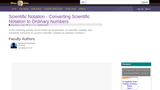Restricted Use
Rating
0.0 stars

The student reads an explanation of scientific notation and performs exercises to convert scientific notation to ordinary numbers.

Subject:
Mathematics
Material Type:
Interactive
Provider:
Wisc-Online
12/23/2015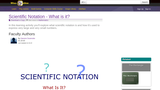Restricted Use
Rating
0.0 stars

In this lesson, scientific notation is explained and demonstrated.

Subject:
Mathematics
Material Type:
Interactive
Provider:
Wisc-Online
12/23/2015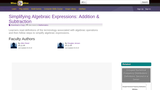Restricted Use
Rating
0.0 stars

Learners read definitions of the terminology associated with algebraic operations and then follow steps to simplify algebraic expressions.

Subject:
Mathematics
Material Type:
Interactive
Provider:
Wisc-Online
12/23/2015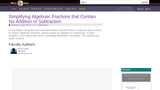Restricted Use
Rating
0.0 stars

In this highly interactive and animated object, learners follow a step-by-step process to reduce algebraic fractions, which contain no addition or subtraction, to their simplest, most elementary form. Immediate feedback is provided. This activity has audio content.

Subject:
Mathematics
Material Type:
Interactive
Provider:
Wisc-Online
12/23/2015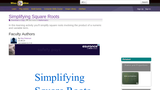Restricted Use
Rating
0.0 stars

The student simplifies square roots involving the product of a numeric and variable term.

Subject:
Mathematics
Material Type:
Interactive
Provider:
Wisc-Online
12/23/2015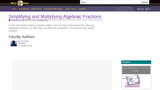Restricted Use
Rating
0.0 stars

In this interactive object, learners follow step-by-step instructions for reducing algebraic fractions so that they can then be multiplied. This activity has audio content.

Subject:
Mathematics
Material Type:
Interactive
Provider:
Wisc-Online
12/23/2015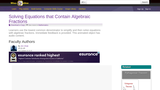Restricted Use
Rating
0.0 stars

Learners use the lowest common denominator to simplify and then solve equations with algebraic fractions. Immediate feedback is provided. This animated object has audio content.

Subject:
Mathematics
Material Type:
Interactive
Provider:
Wisc-Online
12/23/2015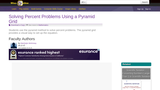Restricted Use
Rating
0.0 stars

Students use the pyramid method to solve percent problems. The pyramid grid provides a visual way to set up the equation.

Subject:
Mathematics
Material Type:
Interactive
Provider:
Wisc-Online
12/23/2015Restricted Use
Rating
0.0 stars

Learners study the properties of a sphere and use geometric formulas to find volume and surface area.

Subject:
Mathematics
Material Type:
Interactive
Provider:
Wisc-Online
12/23/2015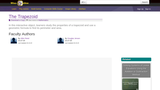Restricted Use